## Creating a compound path with gradient mesh

Some shapes, no matter how hard you try, won’t let you apply a gradient mesh. The following steps should be used if you want to apply a gradient mesh to a compound path.

## Step 1

Let us build a Gradient Mesh for the Compound Path shown in the picture below. Gradient Mesh can’t be applied to Compound Paths using the regular techniques, we will have to build one.## Step 2

Start creating the Gradient Mesh, we will do this by transforming a Rectangle. Lock the sub-layer containing the original shape, this will be used as a template. Draw a Rectangle (as shown below) and change its opacity to 50%.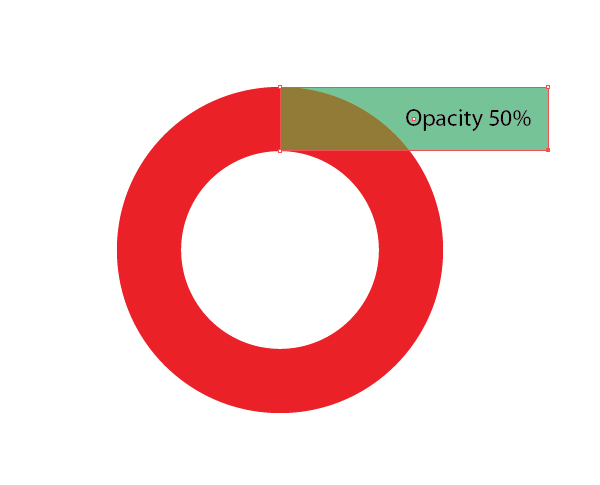## Step 3

Select the rectangle and go to Object > Create Gradient Mesh, set the values as shown. The mesh should be as simple as possible, the number of columns must equal the number of bends, so the circumference has 4.## Step 4

Let’s transform the rectangle. We will be using the following tools: the Lasso Tool (Q); Direct Selection Tool (A); Rotate Tool (R). Select the Lasso Tool (Q). Select anchor points, as shown in the figure below.## Step 5

Take the Direct Selection Tool (A) and move the selected segments to the first “bend” of the guide shape.## Step 6

Take the Rotate Tool (R) and place the axis of rotation on point “A” and rotate the segment 90 degrees while holding down the Shift key.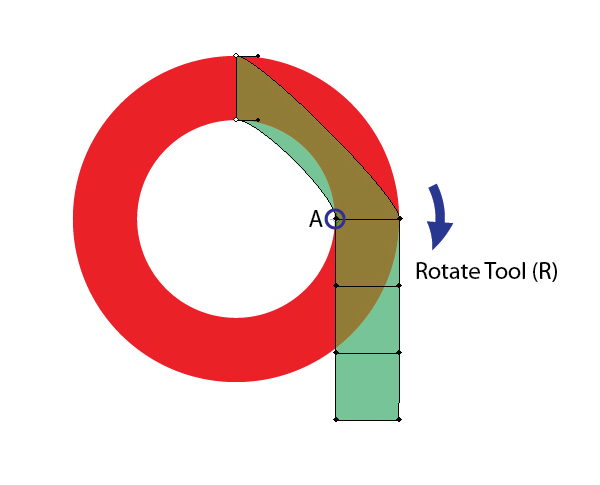## Step 7

Using this technique, repeat the same step for the remainder of the segments.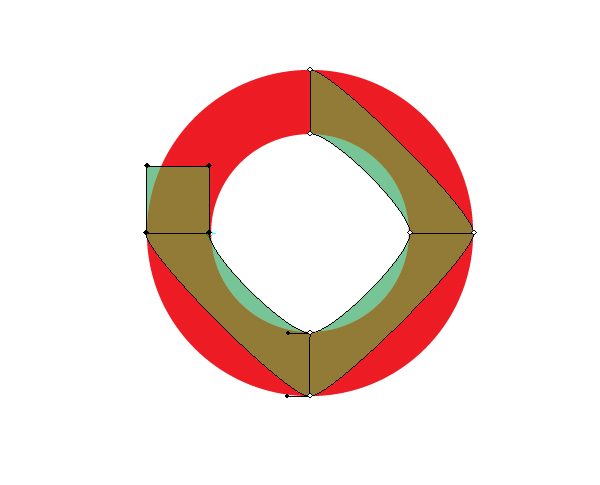## Step 8

Adjusting the handles of the anchor points will improve the contour of your shape. Hold the Shift key while moving the handles to keep them straight.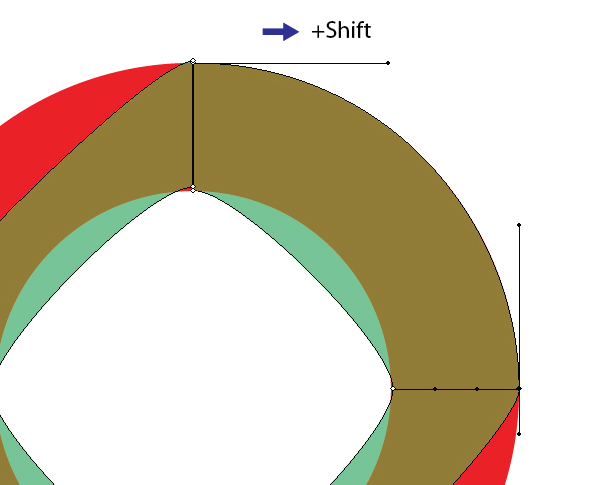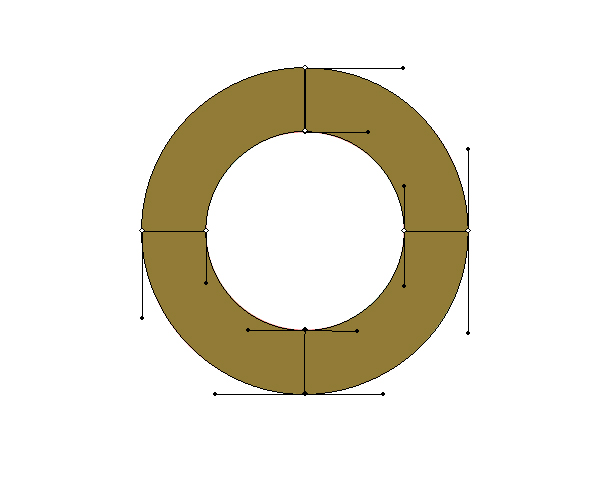## Step 9

Remove the template, change the opacity to 100% and, if needed, add new points to the mesh using the Mesh Tool (U).(via @vectortuts)

•